Ex 5.1

Chapter 5 Class 12 Continuity and Differentiability
Serial order wise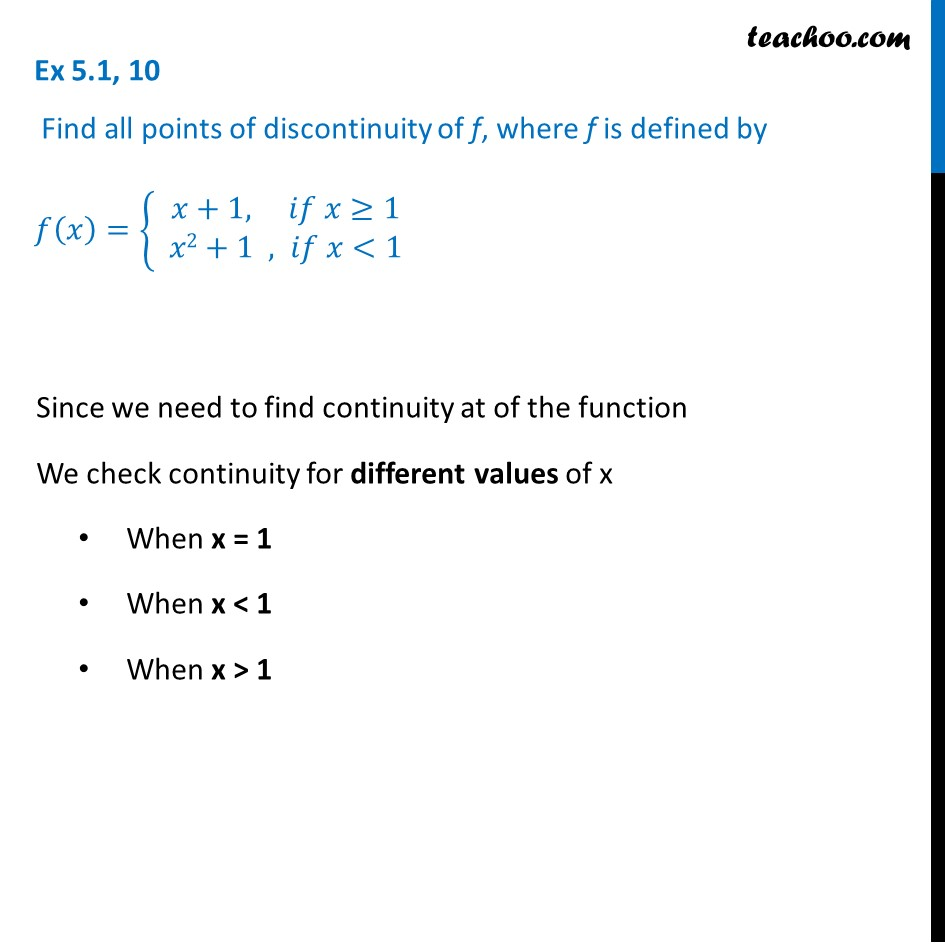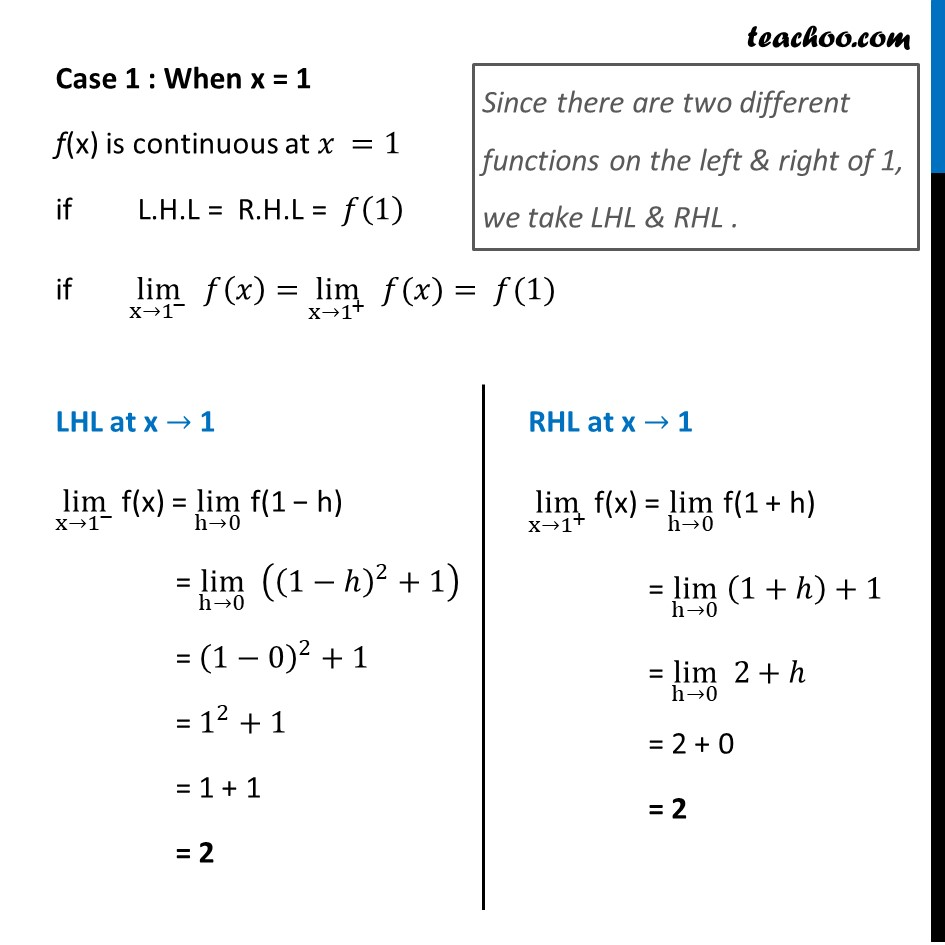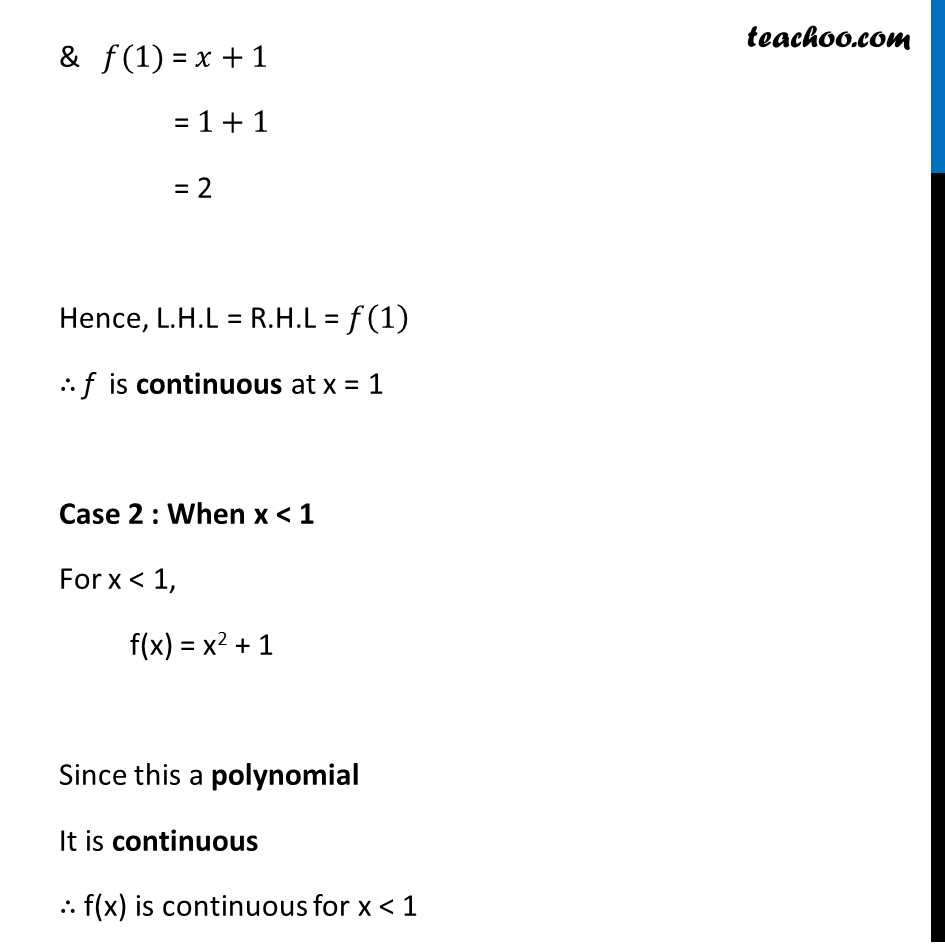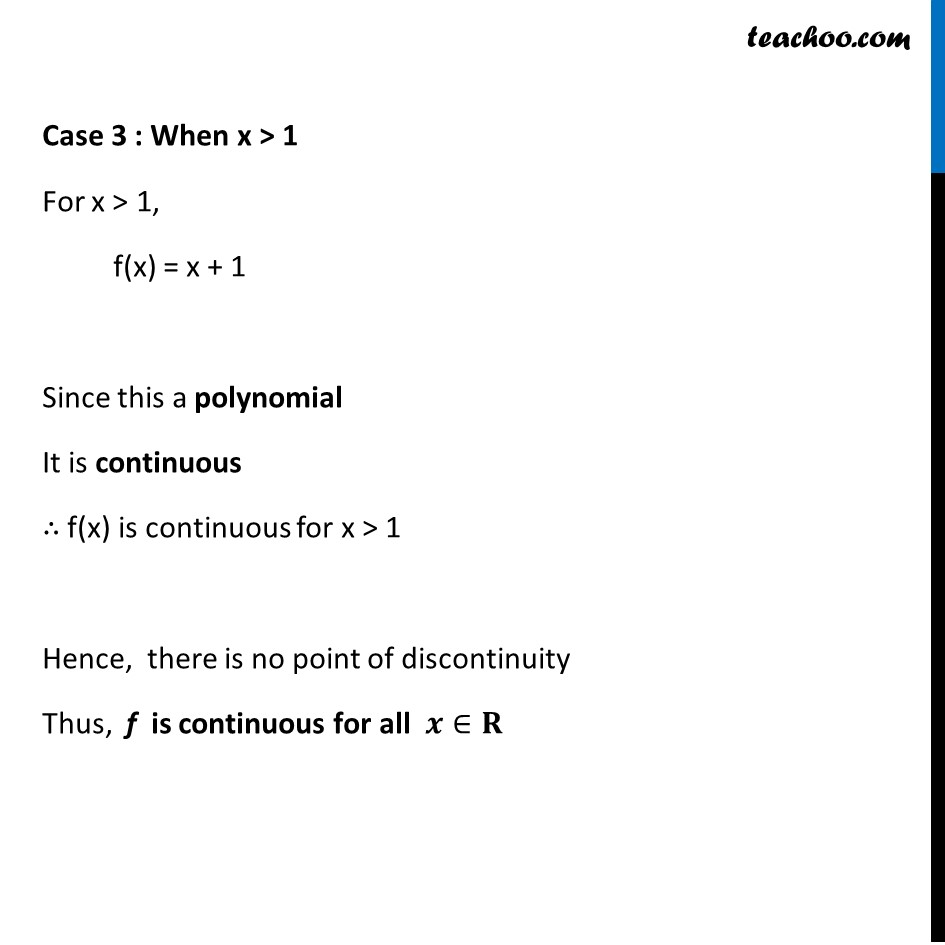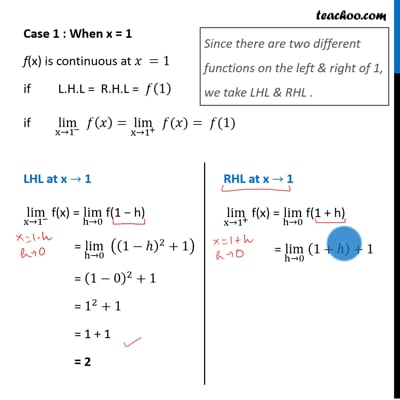This video is only available for Teachoo black users

Solve all your doubts with Teachoo Black (new monthly pack available now!)

### Transcript

Ex 5.1, 10 Find all points of discontinuity of f, where f is defined by 𝑓(𝑥)={ █(𝑥+1, 𝑖𝑓 𝑥≥1@&𝑥2+1 , 𝑖𝑓 𝑥<1)┤ Since we need to find continuity at of the function We check continuity for different values of x When x = 1 When x < 1 When x > 1 Case 1 : When x = 1 f(x) is continuous at 𝑥 =1 if L.H.L = R.H.L = 𝑓(1) if lim┬(x→1^− ) 𝑓(𝑥)=lim┬(x→1^+ ) " " 𝑓(𝑥)= 𝑓(1) Since there are two different functions on the left & right of 1, we take LHL & RHL . LHL at x → 1 lim┬(x→1^− ) f(x) = lim┬(h→0) f(1 − h) = lim┬(h→0) ((1−ℎ)^2+1) = (1−0)^2+1 = 1^2+1 = 1 + 1 = 2 RHL at x → 1 lim┬(x→1^+ ) f(x) = lim┬(h→0) f(1 + h) = lim┬(h→0) (1+ℎ)+1 = lim┬(h→0) 2+ℎ = 2 + 0 = 2 & 𝑓(1) = 𝑥+1 = 1+1 = 2 Hence, L.H.L = R.H.L = 𝑓(1) ∴ f is continuous at x = 1 Case 2 : When x < 1 For x < 1, f(x) = x2 + 1 Since this a polynomial It is continuous ∴ f(x) is continuous for x < 1 & 𝑓(1) = 𝑥+1 = 1+1 = 2 Hence, L.H.L = R.H.L = 𝑓(1) ∴ f is continuous at x = 1 Case 2 : When x < 1 For x < 1, f(x) = x2 + 1 Since this a polynomial It is continuous ∴ f(x) is continuous for x < 1 Case 3 : When x > 1 For x > 1, f(x) = x + 1 Since this a polynomial It is continuous ∴ f(x) is continuous for x > 1 Hence, there is no point of discontinuity Thus, f is continuous for all 𝒙∈𝐑